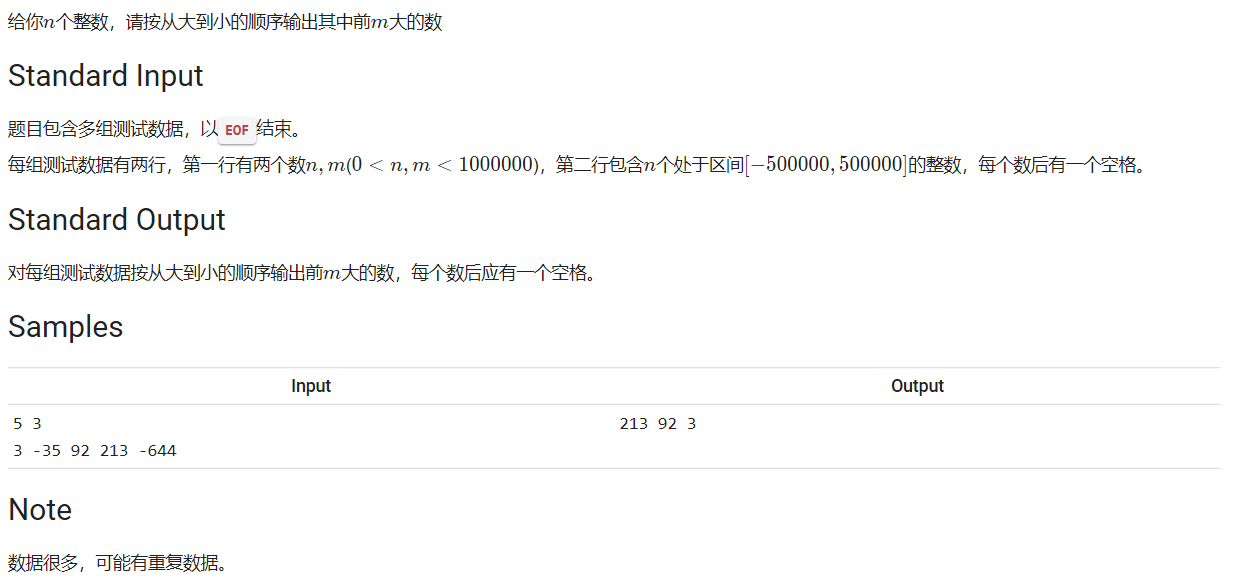• ## 哈希排序算法

千次阅读 2020-03-05 11:27:39
将数的大小映射到数组下标，下标越大，这个数越大，处理数组的数据实现。具体的数有多大，这个数组的范围就要开到多大，所以一定要仔细审题，理清题意中的范围。 细节见代码注释… 题目描述 TimeLimit:10...
哈希排序 遇到这样一道题，数据很大，如果将数字排序后再输出，得到的结果是TLE（超时）时间复杂度

O

(

n

2

)

.

O(n^2).

我们选择用哈希排序的方法，降低时间复杂度到

O

(

n

)

O(n)

，同时也牺牲了空间【用空间换取时间】。 将数的大小映射到数组下标，下标越大，这个数越大，处理数组的数据实现。具体的数有多大，这个数组的范围就要开到多大，所以一定要仔细审题，理清题意中的范围。 细节见代码注释… 题目描述T

i

m

e

L

i

m

i

t

:

1000

m

s

Time Limit : 1000ms

#include<bits/stdc++.h>
#define N 1000000
using namespace std;
int h[N];//N很大，声明全局数组
//哈希算法，将数的大小映射到数组下标，下标越大，这个数越大，处理数组的数据实现
int main() {
int n,m,x;
//int T;
//cin>>T;
while(scanf("%d%d",&n,&m)==2){//以EOF文件结束符结束
//cin>>n>>m;
int i,j;
memset(h,0,sizeof(h));//循环内清零
for(i=0;i<n;i++){
cin>>x;
h[x+N/2]++;//把-500000~500000映射到0~1000000并作为数组下标
}
int t=0;//用来计数，只输出m个
for(i=N-1;i>=0;i--){
for(j=0;j<h[i];j++){//相同的数输出h[i]次
cout<<i-N/2<<" ";
t++;
if(t==m) break;//退到上一层循环
}
if(t==m) break;//再退一次哦
}
cout<<endl;
}
}


展开全文• JS排序算法：冒泡法、快速排序法、选择排序法、插入排序法哈希排序//生成数组 var arr = new Array(1000); for (var i = 0; i ; i++) { arr[i] = (Math.round(Math.random() * 1000)); }1.冒泡法 排序思想：...
JS排序算法：冒泡法、快速排序法、选择排序法、插入排序法、哈希排序
//生成数组
var arr = new Array(1000);
for (var i = 0; i < 1000; i++) {
arr[i] = (Math.round(Math.random() * 1000));
}
1.冒泡法  排序思想：数组相邻两项进行比较，如果前一项比后一项大则交换位置，比较arr.length-1轮,每一轮把最大的一位数放最后
//冒泡法排序
function sortArr(arr) {
for (var i = 0; i < arr.length - 1; i++) {//比较arr.length-1轮
for (var j = i + 1; j < arr.length; j++) {
if (arr[i] > arr[j]) {//交换
var temp = arr[i];//临时变量
arr[i] = arr[j];
arr[j] = temp;

}
}
}
return arr;
}
2.快速排序法  原理：通过一趟排序将要排序的数据分割成独立的两部分，其中一部分的所有数据都比另外一部分的所有数据都要小，然后再按此方法对这两部分数据分别进行快速排序，  整个排序过程可以递归进  （1）在数据集之中，选择一个元素作为”基准”（pivot）。  （2）所有小于”基准”的元素，都移到”基准”的左边；所有大于”基准”的元素，都移到”基准”的右边。  （3）对”基准”左边和右边的两个子集，不断重复第一步和第二步，直到所有子集只剩下一个元素为止。  快速排序的最坏运行情况是O(n²)，比如说顺序数列的快排。但它的平摊期望时间是O(n log n) ，且O(n log n)记号中隐含的常数因子很小，比复杂度稳定等于O(n log n)的归并排序要小很多。所以，对绝大多数顺序性较弱的随机数列而言，快速排序总是优于归并排序。
//快速排序法
function sortQuick(arr) {
if (arr.length <= 1) {//递归结束判断条件
return arr;
} else {
var index = Math.floor(arr.length / 2);//取最中间的那个元素
var len = arr.splice(index, 1);
var left = [];
var right = [];

for (var i = 0; i < arr.length; i++) {
if (arr[i] < len) {
left.push(arr[i]);
} else {
right.push(arr[i]);
}
}
return sortQuick(left).concat(len, sortQuick(right));
}

}
3.选择排序法  原理：每一次从待排序的数据元素中选出最小（或最大）的一个元素，存放在序列的起始位置，直到全部待排序的数据元素排完。 选择排序是不稳定的排序方法  假定数组每次比较范围内第一个元素最小min，和剩下的比较，如果比假定的这个元素小，则令min为这个元素，直到找到最小的，然后交换位置,每比较一次，  就把最小的一位数找出来放数组最前面。
//选择排序
function sortSelect(arr) {
for (var i = 0; i < arr.length; i++) {
var min = arr[i];
var index = i;
for (var j = i + 1; j < arr.length; j++) {
if (arr[j] < min) {
min = arr[j];
index = j;
}
}
if (index != i) {
var temp = arr[i];
arr[i] = arr[index];
arr[index] = temp;
}

}

}
4.插入排序法  插入算法把要排序的数组分成两部分：第一部分包含了这个数组的所有元素，但将最后一个元素除外（让数组多一个空间才有插入的位置），  而第二部分就只包含这一个元素（即待插入元素）。在第一部分排序完成后，再将这个最后元素插入到已排好序的第一部分中。
//插入排序
function sortInsert(arr) {
for (var i = 0; i < arr.length - 1; i++) {
var insert = arr[i + 1];
var index = i+1;
for(var j=i;j>=0;j--){
if(insert > arr[j]){
arr[j+1] = arr[j];
index = j;
}
}
arr[index] = insert;
}

}
5.哈希排序  原理：先将整个待排元素序列分割成若干个子序列（由相隔某个“增量”的元素组成的）分别进行直接插入排序，然后依次缩减增量再进行排序，待整个序列中的元素基本有序  （增量足够小）时，再对全体元素进行一次直接插入排序。
//哈希排序
function sortShell(arr){
var len = arr.length,
temp,
gap = 1;
while(gap < len/3){
gap = gap*3+1;
}
for(gap;gap>0;gap = Math.floor(gap/3)){
for(var i=gap;i<len;i++){
temp = arr[i];
for(var j=i-gap;j>0&&arr[j]>temp;j-=gap){
arr[j+gap] = arr[j];
}
arr[j+gap] = temp;
}
}
return arr;
}

展开全文javascript 插入排序 快速排序 冒泡排序
• 哈希排序算法(Hash)，是目前我认为速度最快的排序算法之一，时间复杂度为O(n)，而且我认为很简单。它的主体思路是：定义一数组，每元素表示它的下标在数列中的个数，最后用循环完成排序。 例如给你一上限不...

哈希排序算法(Hash)，是目前我认为速度最快的排序算法之一，时间复杂度为O(n)，而且我认为很简单。它的主体思路是：定义一个数组，每个元素表示它的下标在数列中的个数，最后用循环完成排序。
例如给你一个上限不超过100的数列，要求你从小到大进行排序。这时我们就可以用哈希排序算法，代码如下。

#include<cstdio>
int a;
int main()
{
int n;
scanf("%d",&n);
int i,j,t;
for(i=1;i<=n;i++)
{
scanf("%d",&t);
a[t]++;
}
for(i=0;i<100;i++) for(j=0;j<a[i];j++) printf("%d",i);
}

转载于:https://www.cnblogs.com/xiaoshenWXY/p/4646797.html
展开全文• ## 哈希排序

千次阅读 2019-09-01 13:01:24
哈希排序应该要和希尔排序区分开来，哈希排序的思想很简单的，典型的以空间换取时间的排序算法，其时间复杂度可以做到O(n)。简单来说就是打表，用数组下标来对应数值，用值来记录个数。具体实现看下面例子： #...
哈希排序应该要和希尔排序区分开来，哈希排序的思想很简单的，典型的以空间换取时间的排序算法，其时间复杂度可以做到O(n)。简单来说就是打表，用数组下标来对应数值，用值来记录个数。具体实现看下面例子：
#include<bits/stdc++.h>
using namespace std;
int main()
{
int a[]={3,8,67,45,11,0,34,67,23,8} ;
int arr;//注意开辟的数组大小要超过排序数字的最大值
memset(arr,0,sizeof(arr));

for(int i=0;i<10;i++)
arr[a[i]]++;

for(int i=0;i<100;i++)
{
while(arr[i]--)
{
cout<<i<<" ";
}
}

return 0;
} 
注意：开辟的数组下标最大要大于排序数字；
其实负数也能够排序，比如你看：
#include<bits/stdc++.h>
using namespace std;
const int maxs = 200;

int main()
{
int a[]={3,-8,-67,45,11,0,34,-67,23,-8} ;
int arr[maxs];//注意开辟的数组大小要超过排序数字的最大值
memset(arr,0,sizeof(arr));

for(int i=0;i<10;i++)
arr[100+a[i]]++;   //注意加的数要够，不能让下标为负数，也不能超过数组最大小标

for(int i=0;i<maxs;i++)
{
while(arr[i]--)
{
cout<<i-100<<" ";
}
}

return 0;
} 
这种算法也经常用来去重复，但是对于某些刁钻的数据也不太好用。
展开全文哈希 排序
• 哈希排序： ==该方法实质上是一种分组插入方法比较相隔较远距离（称为增量）的，使得移动时能跨过多元素，则进行一次比 较就可能消除多元素交换。D.L.shell于1959年在以他名字命名的排序算法中实现了这...
• #include &lt;iostream&gt; #include &...#define N 10 using namespace std; //声明建大顶堆函数 void BuildMaxHeap(int * array); //声明堆排序函数 void HeapSort(int * array); //声明调...
• 哈希排序详细介绍+案例分析 哈希表（Hash table，也叫散列表），是根据关键码值(Key value)而直接进行访问的数据结构。也就是说，它通过把关键码值映射到表中一位置来访问记录，以加快查找的速度。这映射函数...
• <html> <script> (function main() { var array = [1, 4, -1, 2, 0, -5, -3, 3, -2, -4, 5]; var minusArray = [], positiveArray = []; for( var i = 0; i < array.leng...
• 有效的字母异位词（排序法哈希表法 → 空间优化）242. 有效的字母异位词思路1：排序思路2：使用map 242. 有效的字母异位词 题目链接：https://leetcode-cn.com/problems/valid-anagram/ 解题思路类似：剑指offer...
• 在本文中，提出了一种算法，称为top k RHS（Rank Hash相似度），其中设计了一种排序损失函数来学习哈希函数。 假设哈希函数由1二进制分类器组成。 学习哈希函数的问题可以表述为学习二进制分类器的任务。 该算法...研究论文
• 作者：王为涛最近无聊时，就想起来大学想做的个哈希排序算法，就又按哈希的思想做了优化版的排序算法，请大家指教。注： 写的时候没有参考其他的排序算法，如果有意外和某些已发表的算法重和的话，还请告知，我做...数据结构 线性复杂度 二叉树
• 题目要求 :算出给出的n个数中最大的前m个数，按从大到小排序。 1冒泡排序 时间复杂度O(n^2)，好处，不在占用额外内存。 #include<bits/stdc++.h> using namespace std; int a; int n,m; void swap(int......